## POLYRING

 Creates a polygon ring image from one or more images.

 USAGE: polyring [-d duplicates] [-f format] [-b bcolor] [-m maxsize] infile1 [infile2 infile3 ... ] outfile -d ... duplicates ... duplicate images to use when only one input image is supplied, ..................... including the input; integer>=3; default=6; the associated ..................... polygon number of sides will be the value of duplicates; ..................... if multiple images are supplied, then that will take precedence; ..................... if more than one image is supplied, then there must be at ..................... minimum 3 images supplied and no more than 6 images supplied -f ... format ....... format for the output; polygon or ring; default=polygon -b ... bcolor ....... color to use for the background color of the output; ..................... default=white -m ... maxsize ...... output image's maximum size; integer>0; default is twice the ..................... input image's minimum width plus twice the minimum height plus ..................... twice computed radius PURPOSE: To create a polygon ring from one or more images. DESCRIPTION: POLYRING creates a polygon ring image from one or more images. One input may be duplicated multiple times so that there are 3 or more copies. Or 3 or more different input images may be supplied. If multiple input images are supplied, they will be center cropped to the minimum dimensions from all the images supplied. ARGUMENTS: -d duplicates ... DUPLICATES is the number of duplicate images to use when only one input image is supplied, including the input. Values are integer>=3. The default=6. The associated polygon number of sides will be the value of duplicates. If multiple images are supplied, then that will take precedence. If more than one image is supplied, then there must be at minimum 3 images supplied and no more than 6 images supplied. -f format ... FORMAT for the output. Choices are: polygon (p) or ring (r). The default=polygon. The polygon shape will be determined by the total number of images used, including the duplicates. -b bcolor ... BCOLOR is the background color of the output. Any valid IM color is allowed. The default=white. -m maxsize ... MAXSIZE is the output image's maximum size (dimension). The value is an integer>0. The default is twice the input image's minimum width plus twice the minimum height plus twice computed radius. CAVEAT: No guarantee that this script will work on all platforms, nor that trapping of inconsistent parameters is complete and foolproof. Use At Your Own Risk.

### EXAMPLES

 Example 1 OriginalArguments: -d 3 -f polygon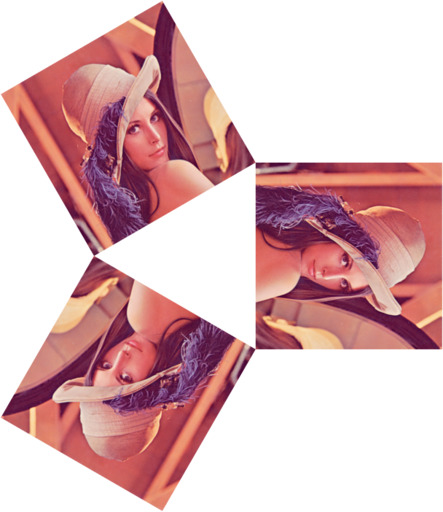Arguments: -d 4 -f polygon -m 512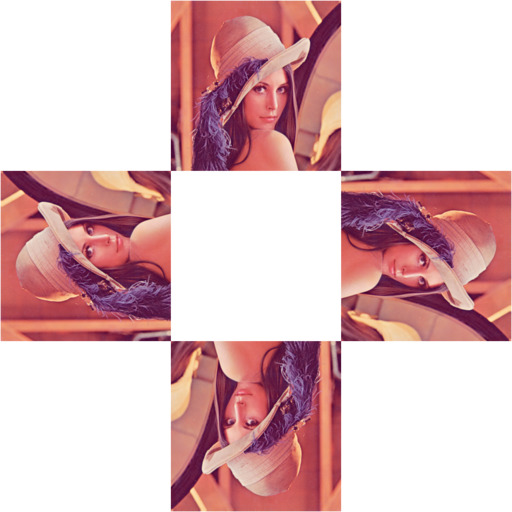Arguments: -d 5 -f polygon -m 512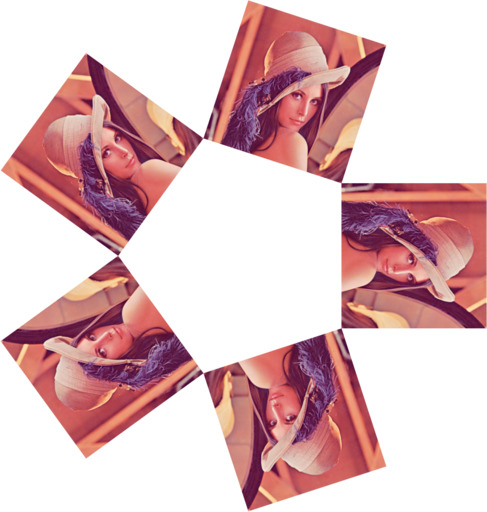Arguments: -d 6 -f polygon -m 512Arguments: -d 8 -f polygon -m 512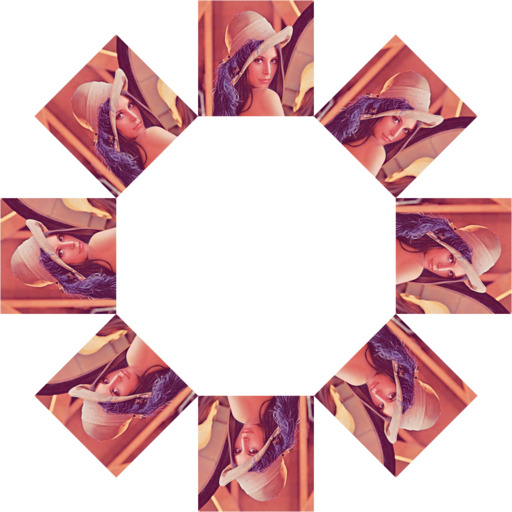Arguments: -d 6 -f ring -m 512Example 2 Originals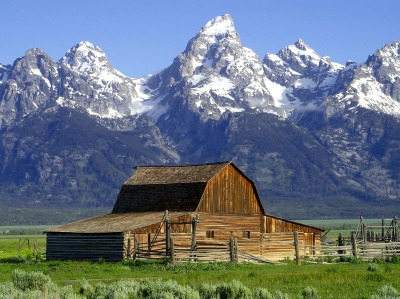Arguments: -f polygon -m 512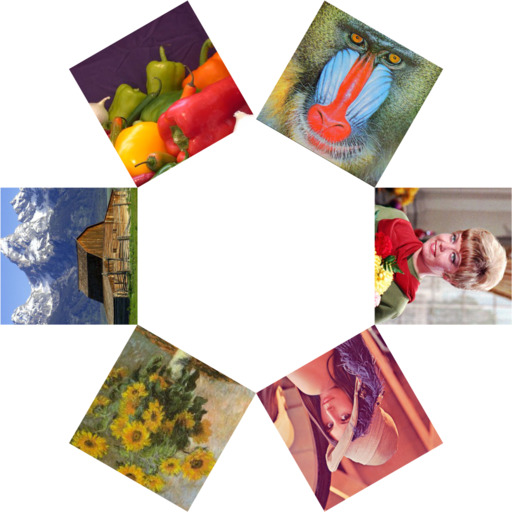Arguments: -f ring -m 512What the script does is as follows for polygon method: read image compute the anglular increment for the number of duplicates provided compute the x and y offset to the center of the input compute the appropriate radius so that the bottoms of the duplicates just touch at the radius loop over the input for the number of duplicates compute the rotation angle for the position on the circle of the given radius compute the rotation of the image use distort SRT to rotate and offset the image use -layers merge to merge all the processed duplicates write the output This is equivalent to the following IM commands number=\$duplicates angle=\$(convert xc: -format "%[fx:360/\$number]" info:) ix=\$(convert xc: -format "%[fx:\$width/2]" info:) iy=\$(convert xc: -format "%[fx:\$height/2]" info:) radius=\$(convert xc: -format "%[fx:(\$height/2) + \$width/(2*tan((pi/180)*(\$angle/2)))]" info:) for ((i=1; i<=number; i++)); do rot1=\$(convert xc: -format "%[fx:\$i*\$angle]" info:) rot2=\$(convert xc: -format "%[fx:\$rot1+90]" info:) ox=\$(convert xc: -format "%[fx:(\$radius+\$width)+\$radius*cos((pi/180)*\$rot1)]" info:) oy=\$(convert xc: -format "%[fx:(\$radius+\$height)+\$radius*sin((pi/180)*\$rot1)]" info:) convert "\$infile" -virtual-pixel none +distort SRT "\$ix,\$iy 1 \$rot2 \$ox,\$oy" \$dir/tmp_\$i.miff done convert \$dir/tmp_*.miff -background "\$bcolor" -layers merge +repage \$resizing "\$outfile"